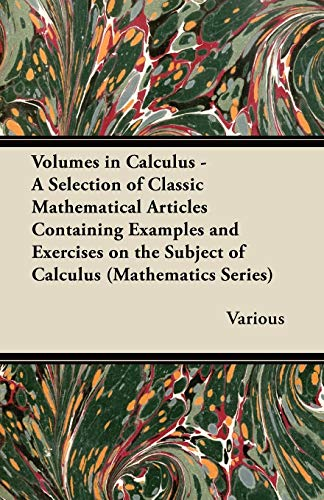• Free Shipping on all orders in Australia
• Over 7 million books in stock
• Proud to be B-Corp
• We aim to be carbon neutral by 2022
• Over 120,000 Trustpilot reviews
Item 1 of 0# Volumes in Calculus - A Selection of Classic Mathematical Articles Containing Examples and Exercises on the Subject of Calculus (Mathematics Series) by Various

Condition - New
\$35.09
Only 2 left

## Volumes in Calculus - A Selection of Classic Mathematical Articles Containing Examples and Exercises on the Subject of Calculus (Mathematics Series) Summary

### Volumes in Calculus - A Selection of Classic Mathematical Articles Containing Examples and Exercises on the Subject of Calculus (Mathematics Series) by Various

Unfortunately we do not have a summary for this item at the moment

NLS9781447457008
9781447457008
1447457005
Volumes in Calculus - A Selection of Classic Mathematical Articles Containing Examples and Exercises on the Subject of Calculus (Mathematics Series) by Various
New
Paperback